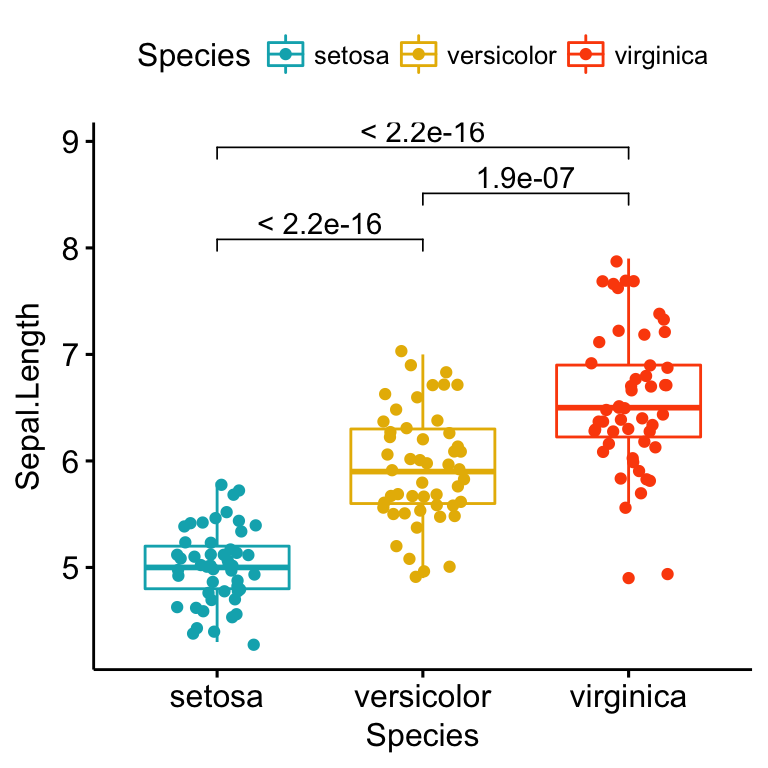# Articles - R Graphics Essentials

This book presents the essentials of R graphics systems to create to quickly create beautiful plots using either R base graphs or ggplot2.

You 'll learn:

- How to easily create and customize beautiful graphics using ggplot2 packages and R base functions

- Type of graphics for visualizing categorical and continuous variables

- How to add automatically p-values to box plots, bar plots and alternatives

- How to add marginal density plots and correlation coefficients to scatter plots

- Key methods for analyzing and visualizing multivariate data

- R functions and packages for plotting time series data

- How to combine multiple plots on one page to create production-quality figures.

Sort by

## R Basics for Data Visualization

By , The in R Graphics EssentialsR is a free and powerful statistical software for analyzing and visualizing data. In this chapter, you’ll learn: the basics of R programming for importing and manipulating your data: filtering... [Read more]

## Plot One Variable: Frequency Graph, Density Distribution and More

By , The in R Graphics EssentialsTo visualize one variable, the type of graphs to use depends on the type of the variable: For categorical variables (or grouping variables). You can visualize the count of categories using a... [Read more]

## Plot Grouped Data: Box plot, Bar Plot and More

By , The in R Graphics EssentialsIn this chapter, we’ll show how to plot data grouped by the levels of a categorical variable. We start by describing how to plot grouped or stacked frequencies of two categorical variables. This... [Read more]

## Plot Two Continuous Variables: Scatter Graph and Alternatives

By , The in R Graphics EssentialsScatter plots are used to display the relationship between two continuous variables x and y. In this article, we’ll start by showing how to create beautiful scatter plots in R. We’ll use helper... [Read more]

## Plot Multivariate Continuous Data

By , The in R Graphics EssentialsWhen you have a bivariate data, you can easily visualize the relationship between the two variables by plotting a simple scatter plot. For a data set containing three continuous variables, you... [Read more]

## Visualizing Multivariate Categorical Data

By , The in R Graphics EssentialsTo visualize a small data set containing multiple categorical (or qualitative) variables, you can create either a bar plot, a balloon plot or a mosaic plot. For a large multivariate... [Read more]

## Plot Time Series Data Using GGPlot

By , The in R Graphics EssentialsIn this chapter, we start by describing how to plot simple and multiple time series data using the R function geom_line() [in ggplot2]. Next, we show how to set date axis limits and add... [Read more]

## GGPlot Facet: Quick Reference

By , The in R Graphics EssentialsThis chapter provides a quick reference to facet_wrap() and facet_grid() for faceting a ggplot into multiple panels. Facets divide a ggplot into subplots based on the values of one or more... [Read more]

## Combine Multiple GGPlots in One Graph

By , The in R Graphics EssentialsThis chapter describes, step by step, how to combine multiple ggplots in one graph, as well as, over multiple pages, using helper functions available in the ggpubr R package. We’ll also... [Read more]

## GGPlot Cheat Sheet for Great Customization

By , The in R Graphics EssentialsThis chapter provides a cheat sheet to change the global appearance of a ggplot. You will learn how to: Add title, subtitle, caption and change axis labels Change the appearance - color,... [Read more]